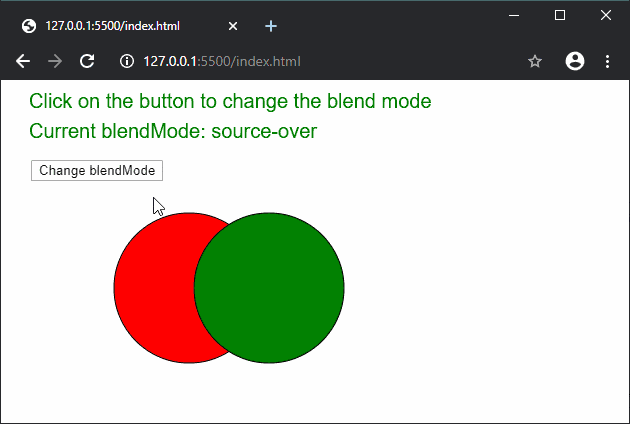# p5.js | blendMode() Function

• Last Updated : 20 Feb, 2020

The blendMode() function is used to blend two pixels according to the given blending mode. The different types of blending modes have different methods of mixing the source pixels with the ones present in the display window, to produce the resulting pixel.

Syntax:

`blendMode( mode )`

Parameters: This function accepts single parameter mode that is used to blend the pixels. It can have the following values:

• BLEND: It blends the pixels using linear interpolation of the colors. It is the default blending mode.
• ADD: It produces the new color by adding the colors of both the pixels.
• DARKEST: It uses only the darker color of the two pixels.
• LIGHTEST: It uses only the lighter color of the two pixels.
• DIFFERENCE: It subtracts colors from the underlying image.
• EXCLUSION: It has a similar effect to the “difference” property with less intensity.
• MULTIPLY: It multiplies both the colors resulting in a darker image.
• SCREEN: It has the opposite effect to the “multiply” effect and uses inverse values of the colors.
• REPLACE: It entirely replaces the pixels of the first with the pixels of the other while ignoring the alpha values.
• REMOVE: It removes the pixels from the second color using its alpha strength.
• OVERLAY: It is a mix of the “multiply” and “screen” modes. It multiplies the light values and screens the dark values. It works only in the 2D renderer.
• HARD_LIGHT: It applies the “screen” effect when the gray value is above 50% and “multiply” when it is lower. It works only in the 2D renderer.
• SOFT_LIGHT: It is a mix of “darkest” and “lightest”. It works like the “overlay” mode with less intensity. It works only in the 2D renderer.
• DODGE: It lightens the light tones and increases the contrast, while ignoring the dark tones. It works only in the 2D renderer.
• BURN: It lightens the dark tones and increases the contrast, while ignoring the light tones. It works only in the 2D renderer.
• SUBTRACT: It applies the final color based on the remainder of the two pixels. It works only in the WEBGL renderer.

The example below illustrates the blendMode() function in p5.js:

Example:

 `function` `setup() { ` `  ``blendModes = [ ` `    ``BLEND, ` `    ``ADD, ` `    ``DARKEST, ` `    ``LIGHTEST, ` `    ``DIFFERENCE, ` `    ``EXCLUSION, ` `    ``MULTIPLY, ` `    ``OVERLAY, ` `    ``HARD_LIGHT, ` `    ``SOFT_LIGHT, ` `    ``DODGE, ` `    ``BURN ` `  ``] ` ` `  `  ``index = 0; ` `  ``currBlendMode = blendModes[index]; ` ` `  `  ``createCanvas(600, 300); ` `  ``textSize(20); ` `} ` ` `  `function` `draw() { ` `  ``clear(); ` `  ``text(``'Click on the button to change the blend mode'``, 20, 20); ` `  ``text(``"Current blendMode: "` `+ currBlendMode, 20, 50); ` ` `  `  ``btn = createButton(``"Change blendMode"``); ` `  ``btn.position(30, 80); ` `  ``btn.mousePressed(changeBlendMode); ` ` `  `  ``// Set the blend mode ` `  ``blendMode(currBlendMode); ` ` `  `  ``// Draw the first circle ` `  ``fill(``"red"``); ` `  ``circle(180, 200, 150); ` ` `  `  ``// Draw the second circle ` `  ``fill(``"green"``); ` `  ``circle(260, 200, 150); ` `} ` ` `  `function` `changeBlendMode() { ` `  ``if` `(index < blendModes.length - 1) ` `    ``index++; ` `  ``else` `    ``index = 0; ` `  ``currBlendMode = blendModes[index]; ` `} `

Output:Online editor: https://editor.p5js.org/

My Personal Notes arrow_drop_up
Recommended Articles
Page :